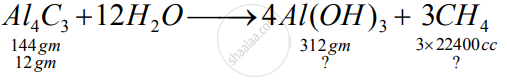Share

# Aluminium Carbide Reacts with Water According to the Following Equation :What Mass of Aluminium Hydroxide is Formed from 12g of Aluminium Carbide? and What Volume of Methane at S.T.P. is Obtained from 12g of Aluminium Carbide? - ICSE Class 10 - Chemistry

ConceptVapour Density and Its Relation to Relative Molecular Mass:

#### Question

Aluminium carbide reacts with water according to the following equation :

Al_4C_3 + 12H_2O-> 4Al(OH)_3 + 3CH_4

1)What mass of aluminium hydroxide is formed from 12g of aluminium carbide?

2) What volume of methane at s.t.p. is obtained from 12g of aluminium carbide?

[Relatively molecular weight of Al_4Cl_3 = 144; Al(OH)_3 = 78]

#### Solution

1)So, the amount of 3 Al(OH)3 formed will be 26 gm

2) From 12 gm Al4C3 5600 cc methane will be formed

Is there an error in this question or solution?

#### APPEARS IN

Solution Aluminium Carbide Reacts with Water According to the Following Equation :What Mass of Aluminium Hydroxide is Formed from 12g of Aluminium Carbide? and What Volume of Methane at S.T.P. is Obtained from 12g of Aluminium Carbide? Concept: Vapour Density and Its Relation to Relative Molecular Mass:.
S